# Arithmetic

Most programs perform arithmetic calculations. Figure 2.9 summarizes the C++ arithmetic operators. Note the use of various special symbols not used in algebra. The asterisk (*) indicates multiplication and the percent sign (%) is the modulus operator that will be discussed shortly. The arithmetic operators in Fig. 2.9 are all binary operators, i.e., operators that take two operands. For example, the expression number1 + number2 contains the binary operator + and the two operands number1 and number2.

Figure 2.9. Arithmetic operators.

C++ operation

C++ arithmetic operator

Algebraic expression

C++ expression

+

f + 7

f + 7

Subtraction

-

p c

p c

Multiplication

*

bm or b · m

b * m

Division

/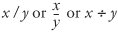x / y

Modulus

%

r mod s

r % s

Integer division (i.e., where both the numerator and the denominator are integers) yields an integer quotient; for example, the expression 7 / 4 evaluates to 1 and the expression 17 / 5 evaluates to 3. Note that any fractional part in integer division is discarded (i.e., truncated)no rounding occurs.

C++ provides the modulus operator, %, that yields the remainder after integer division. The modulus operator can be used only with integer operands. The expression x % y yields the remainder after x is divided by y. Thus, 7 % 4 yields 3 and 17 % 5 yields 2. In later chapters, we discuss many interesting applications of the modulus operator, such as determining whether one number is a multiple of another (a special case of this is determining whether a number is odd or even).

Common Programming Error 2.3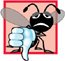Attempting to use the modulus operator (%) with noninteger operands is a compilation error.

Arithmetic Expressions in Straight-Line Form

Arithmetic expressions in C++ must be entered into the computer in straight-line form. Thus, expressions such as "a divided by b" must be written as a / b, so that all constants, variables and operators appear in a straight line. The algebraic notation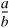is generally not acceptable to compilers, although some special-purpose software packages do exist that support more natural notation for complex mathematical expressions.

Parentheses for Grouping Subexpressions

Parentheses are used in C++ expressions in the same manner as in algebraic expressions. For example, to multiply a times the quantity b + c we write a * (b + c).

Rules of Operator Precedence

C++ applies the operators in arithmetic expressions in a precise sequence determined by the following rules of operator precedence, which are generally the same as those followed in algebra:

1. Operators in expressions contained within pairs of parentheses are evaluated first. Thus, parentheses may be used to force the order of evaluation to occur in any sequence desired by the programmer. Parentheses are said to be at the "highest level of precedence." In cases of nested, or embedded, parentheses, such as

```( ( a + b ) + c )
```

the operators in the innermost pair of parentheses are applied first.

2. Multiplication, division and modulus operations are applied next. If an expression contains several multiplication, division and modulus operations, operators are applied from left to right. Multiplication, division and modulus are said to be on the same level of precedence.
3. Addition and subtraction operations are applied last. If an expression contains several addition and subtraction operations, operators are applied from left to right. Addition and subtraction also have the same level of precedence.

The set of rules of operator precedence defines the order in which C++ applies operators. When we say that certain operators are applied from left to right, we are referring to the associativity of the operators. For example, in the expression

```a + b + c
```

the addition operators (+) associate from left to right, so a + b is calculated first, then c is added to that sum to determine the value of the whole expression. We will see that some operators associate from right to left. Figure 2.10 summarizes these rules of operator precedence. This table will be expanded as additional C++ operators are introduced. A complete precedence chart is included in Appendix A.

Figure 2.10. Precedence of arithmetic operators.

Operator(s)

Operation(s)

Order of evaluation (precedence)

( )

Parentheses

Evaluated first. If the parentheses are nested, the expression in the innermost pair is evaluated first. If there are several pairs of parentheses "on the same level" (i.e., not nested), they are evaluated left to right.

*
/
%

Multiplication
Division
Modulus

Evaluated second. If there are several, they are evaluated left to right.

+
-

Subtraction

Evaluated last. If there are several, they are evaluated left to right.

Sample Algebraic and C++ Expressions

Now consider several expressions in light of the rules of operator precedence. Each example lists an algebraic expression and its C++ equivalent. The following is an example of an arithmetic mean (average) of five terms: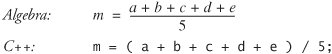The parentheses are required because division has higher precedence than addition. The entire quantity ( a + b + c + d + e ) is to be divided by 5. If the parentheses are erroneously omitted, we obtain a + b + c + d + e / 5, which evaluates incorrectly as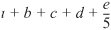The following is an example of the equation of a straight line:No parentheses are required. The multiplication is applied first because multiplication has a higher precedence than addition.

The following example contains modulus (%), multiplication, division, addition, subtraction and assignment operations: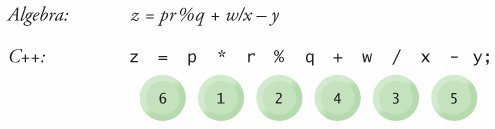The circled numbers under the statement indicate the order in which C++ applies the operators. The multiplication, modulus and division are evaluated first in left-to-right order (i.e., they associate from left to right) because they have higher precedence than addition and subtraction. The addition and subtraction are applied next. These are also applied left to right. Then the assignment operator is applied.

Evaluation of a Second-Degree Polynomial

To develop a better understanding of the rules of operator precedence, consider the evaluation of a second-degree polynomial (y = ax2 + bx + c):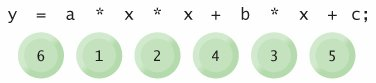The circled numbers under the statement indicate the order in which C++ applies the operators. There is no arithmetic operator for exponentiation in C++, so we have represented x2 as x * x. We will soon discuss the standard library function pow ("power") that performs exponentiation. Because of some subtle issues related to the data types required by pow, we defer a detailed explanation of pow until Chapter 6.

Common Programming Error 2.4Some programming languages use operators ** or ^ to represent exponentiation. C++ does not support these exponentiation operators; using them for exponentiation results in errors.

Suppose variables a, b, c and x in the preceding second-degree polynomial are initialized as follows: a = 2, b = 3, c = 7 and x = 5. Figure 2.11 illustrates the order in which the operators are applied.

Figure 2.11. Order in which a second-degree polynomial is evaluated.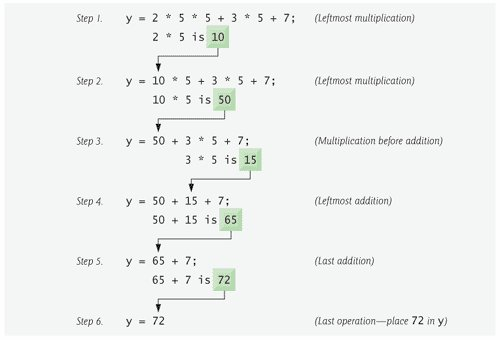As in algebra, it is acceptable to place unnecessary parentheses in an expression to make the expression clearer. These are called redundant parentheses. For example, the preceding assignment statement could be parenthesized as follows:

```y = ( a * x * x ) + ( b * x ) + c;
```

Good Programming Practice 2.14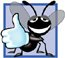Using redundant parentheses in complex arithmetic expressions can make the expressions clearer.C++ How to Program (5th Edition)
ISBN: 0131857576
EAN: 2147483647
Year: 2004
Pages: 627